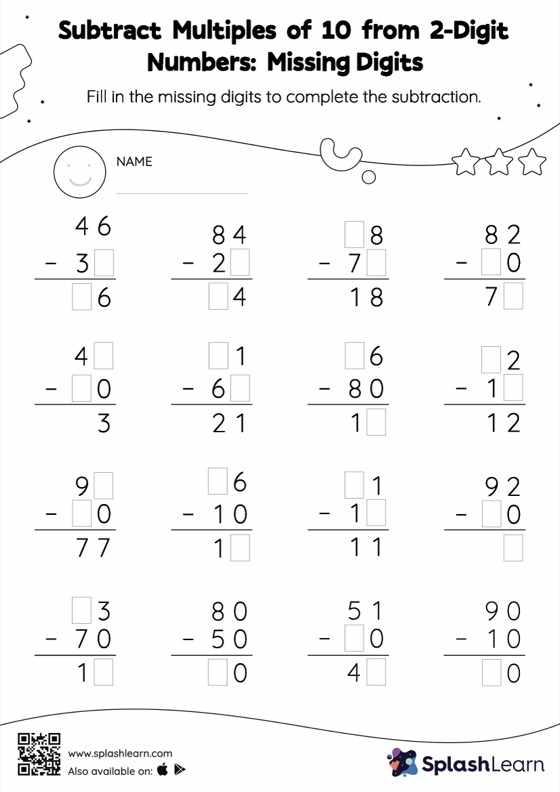# Subtract Multiples of 10 from 2-Digit Numbers: Missing Digits Worksheet

Home > Subtract Multiples of 10 from 2-Digit Numbers: Missing DigitsStudents use the count-back method or the relationship between addition and subtraction to find the missing number if it is a minuend or subtrahend. Also, they subtract the numbers to get the difference if the missing number is the difference. There is a lot of practice with this concept in the worksheet subtract multiples of 10 from 2-digit numbers. As the worksheet uses the column method, it is helpful in getting students toward higher accuracy, especially with bigger numbers and in scenarios where regrouping is required.